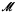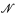Home

# Some highly saturated models of Peano arithmetic

## Extract

Some highly saturated models of Peano Arithmetic are constructed in this paper, which consists of two independent sections. In § 1 we answer a question raised in  by constructing some highly saturated, rather classless models of PA. A question raised in , , ]4] is answered in §2, where highly saturated, nonstandard universes having no bad cuts are constructed.

Highly saturated, rather classless models of Peano Arithmetic were constructed in . The main result proved there is the following theorem. If λ is a regular cardinal andis a λ-saturated model of PA such that ∣M∣ > λ, thenhas an elementary extensionof the same cardinality which is also λ-saturated and which, in addition, is rather classless. The construction in  produced a modelfor which cf() = λ+. We asked in Question 5.1 of  what other cofinalities could such a modelhave. This question is answered here in Theorem 1.1 of §1 by showing that any cofinality not immediately excluded is possible. Its proof does not depend on the theorem from ; in fact, the proof presented here gives a proof of that theorem which is much simpler and shorter than the one in .

Recursively saturated, rather classless κ-like models of PA were constructed in . In the case of singular κ such models were constructed whenever cf(κ) > ℵ0; no additional set-theoretic hypothesis was needed.

Hide All

# Some highly saturated models of Peano arithmetic

## Metrics

### Full text viewsFull text views reflects the number of PDF downloads, PDFs sent to Google Drive, Dropbox and Kindle and HTML full text views.

Total number of HTML views: 0
Total number of PDF views: 0 *Loading metrics...

### Abstract viewsAbstract views reflect the number of visits to the article landing page.

Total abstract views: 0 *Loading metrics...

* Views captured on Cambridge Core between <date>. This data will be updated every 24 hours.

Usage data cannot currently be displayed